cancel
Showing results for
Did you mean:Super User

## YTD expression less one month

Hi All

I have below expression working fine :-

_YTD REV = CALCULATE(CALCULATE(GL[AMOUNT],DATESYTD('Date'[Date],"12/31")), GL[1_REV] = "REV")

Due to GL report alway delay , so i like to modify the above expression one month less. For example now is 1-30 march , the expression will compute YTD from 1 jan till 30 Feb and not 1 jan till 30 march.

Paul

1 ACCEPTED SOLUTIONCommunity Support

YTD Less one Month column is a Flag, please try to build it in your Date table.

In your sample , you build it in another table instead of Date table, so you will get the wrong result.

You table should look like as below. YTD Less one Month column in Jan and Feb in 2021 will return 1 and Mar will return 0.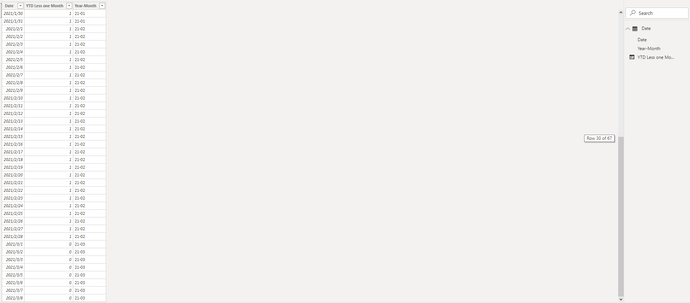Result is as below.

By default: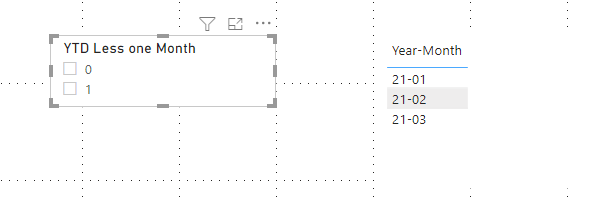Select 1, it will show Jan and Feb in 2021.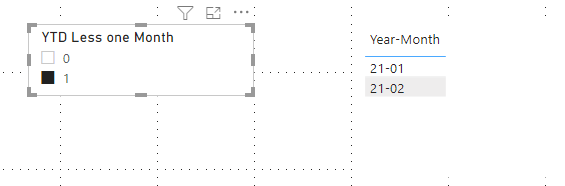Best Regards,

Rico Zhou

If this post helps, then please consider Accept it as the solution to help the other members find it more quickly.

4 REPLIES 4Community Support

I think you want to show values less one month due to report delay.

Try to build a calculated column for filter

``````YTD Less one Month =
IF (
YEAR ( 'Table'[Date] ) = YEAR ( MAX ( 'Table'[Date] ) )
&& MONTH ( 'Table'[Date] )
<= MONTH ( MAX ( 'Table'[Date] ) ) - 1,
1,
0
)``````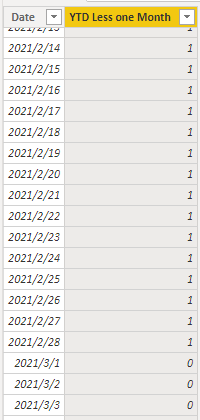Then you can use [YTD Less one Month] = 1 in filter to filter the YTD expression less one month.

And you can show me a screenshot of the result you want to me.

Best Regards,

Rico Zhou

If this post helps, then please consider Accept it as the solution to help the other members find it more quickly.Super User

I have try , i get Jan till DEc 2021. what i expect is getting only Jan till Feb 2021 not include March 2021.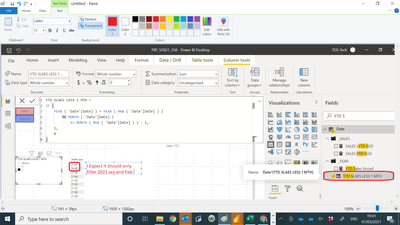Community Support

YTD Less one Month column is a Flag, please try to build it in your Date table.

In your sample , you build it in another table instead of Date table, so you will get the wrong result.

You table should look like as below. YTD Less one Month column in Jan and Feb in 2021 will return 1 and Mar will return 0.Result is as below.

By default:Select 1, it will show Jan and Feb in 2021.Best Regards,

Rico Zhou

If this post helps, then please consider Accept it as the solution to help the other members find it more quickly.Super User

Try like

YTD QTY forced=
var _max = eomonth(today(),-1)
return
if(max('Date'[Date])<=_max, calculate(Sum('order'[Qty]),DATESYTD('Date'[Date])), blank())
//or
//calculate(Sum('order'[Qty]),DATESYTD('Date'[Date]),filter('Date','Date'[Date]<=_max))
//calculate(TOTALYTD(Sum('order'[Qty]),'Date'[Date]),filter('Date','Date'[Date]<=_max))